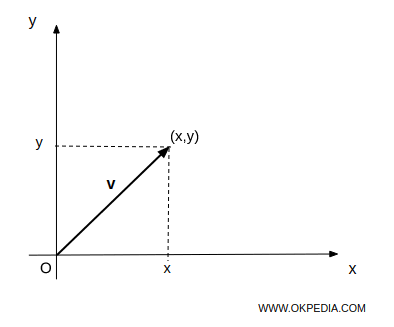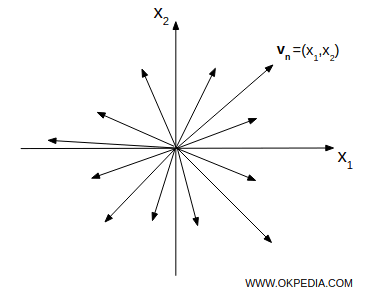# Vector space

A vector space (linear space) is a set whose elements are called vectors.

Two operations are defined in a vector space

In a vector space the vectors may be added together
• Scalar multiplication
In a vector space the vectors may be multiplied by numbers called scalars (e.g. integers, real numbers, complex numbers, etc).

The dimension of a vector space is the number (n) of independent directions in the space.

Example. In a vector space of two dimensions (n=2) the vectors move in two independent directions (x, y axes of the plane).In a three-dimensional vector space (n=3), vectors move in three independent directions (x, y, z axes of space).

A vector space can be finite or infinite dimensional

• finite-dimensional
if dimension is a natural number n∈N
• infinite-dimensional
if dimension is an infinite cardinale n=∞

Each element of the n-dimensional vector space is a vector v = (x1,x2,...,xn) that is a tuple of n real number (vector), where x1,x2,...,xn are called coordinates, elements or components of vector v.The set of all n-tuples of real numbers v = (x1,x2,...,xn) is called n-space and it's denoted by Rn.

Examples. The vector v=(1,2) belong to R2. The vector v=(3,2,1) belong to R3

In a vector space two vectors are equal if the corresponding components are equal.

Examples. The following vectors u=(3,2,1) and v=(3,2,1) are equal. The following vectors u=(3,2,1) and are v=(2,3,1) not equal, because x1u=3≠x1v=2 and x2u=2≠x2v=3

A particular vector is the zero vector

The zero vector is a vector (0,0, ... 0) in which all components are null.

www.okpedia.com - Okpedia - Contact email: info@okpedia.it - P.IVA - 09286581005 - Privacy Google - Disclaimer

Vectors

y